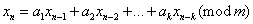ID 772987
Date 12/04/2020
Public

## Uniform Probability Distribution and Basic Pseudo- and Quasi-Random Number Generators

When considering various probability distributions, you should pay special attention to a uniform distribution over a certain interval. Firstly, such a distribution is very convenient for analysis. Secondly, an RNG of uniform distribution can always serve as a basis for an RNG of any other distribution type. That is why this document uses the term basic generators in reference to pseudorandom number generators of uniform distribution.

The observational output sequence of a basic generator should ideally possess the same properties as a sequence of independent variates uniformly distributed over a certain interval. It means that the basic generator should pass various statistical tests for uniformity and independence. It is an a priori fact that the output sequence of such generator is non-random. In other words, a fairly powerful statistical test can always be created for any individual BRNG, which the said generator will definitely fail. However, you should also consider the time required to detect "non-randomness" in the generator. For cryptography, the time required to detect non-randomness may be measured in years of testing conducted on a powerful cluster, while it may be significantly shorter for most of other applications.

Cryptographic RNGs can be slow for other fields. Most of applications benefit from simpler (and faster) generators, such as linear congruential, multiple recursive, feedback-shift-register, or add-with-carry.

Thus, checking the quality of BRNGs requires a "reasonable" set, or battery, of statistical tests. Ideally, you should choose such tests depending on types of problems the generator is intended to solve. A suitable test battery for general-purpose RNG libraries is fairly hard to choose, as the tests it should include are supposed to be versatile and sufficient for many simulation tasks. DIEHARD Battery of Tests by G. Marsaglia [Mars95] is an example of a good set of empirical tests for basic generators. Cryptographic standards such as NIST SP800-22 [NIST800-22] define the requirements including batteries of the tests for non-deterministic random number generators. Still, a specific application type may require a more complete generator testing.

Both empirical testing and theoretical methods are very important for estimating the quality of basic generators:

1. Theoretical evaluation is the first stage in rejecting bad generators. Theoretical research provides the basis for better understanding of generator properties, such as its period length, lattice structure, discrepancy, or equidistribution. The results obtained through theoretical testing refer to a basic generator used over the entire period.

2. Empirical tests ensure that the remaining generators are of acceptable quality by testing a generator over a small fraction of the period actually used.

Good behavior of k-dimensional random number vectors over the entire period indicates (yet does not prove) that similarly good statistical behavior may be observed over a smaller portion of the period [L'Ecu94].

Period of a basic generator is the most important feature that characterizes its quality. For example, one of the VS BRNGs - multiplicative congruential generator MCG31m1 - has a period length of about 231, which can be exhausted within seconds on modern Intel® processors. Taking into account that good statistical behavior of the generator is observed only over a fraction of its period (B.D. Ripley [Ripley87] recommends to take no more than a square root of the period length) you may not consider such period length acceptable. However, in Monte Carlo applications with a relatively small quantity of random numbers to be used, such generators may be useful because of the speed, small memory requirements for keeping the generator state, and efficient methods available for generation of random subsequences. For example, while estimating a global solution to an integral equation through the Monte Carlo method, the same random numbers should be used for different parameters [Mikh2000]. Modern computational capacities require BRNGs of at least 260 period length. All other VS BRNGs meet these requirements.

Pseudorandom number generators are commonly recursive integer sequences in modular arithmetic, for example:Theoretical research looks for such values for parameters k, ai, m that result in good quality properties of the output sequence in terms of period length, lattice structure, discrepancy, equidistribution, and so on. In particular, if m is a prime number selected with proper coefficients ai, a period length of order mk may be obtained. Nevertheless, m is often taken as 2p(p >1) because of efficient modulo m reduction. Some authors do not recommend using m in the form of a power of 2 as the lower bits of the generated random numbers prove to be non-random on the whole. For example, see D. Knuth [Knuth81], P. L’Ecuyer [L'Ecu94]. However, this is irrelevant for most of Monte Carlo applications. Moreover, even if m is a prime number, you should also be careful when selecting random bits in the output sequence.

For the same reasons, quasi-random number generators filling a hypercube as evenly as possible are called in VS Basic Random Number Generators as well. Quasi-random sequences filling a space according to a non-uniform distribution can be generated by transforming a sequence produced by a basic quasi-random number generator. In most cases, tests designed for pseudorandom number generators cannot be used for quasi-random number generators. Special batteries of tests should be designed for basic quasi-random number generators.